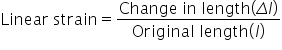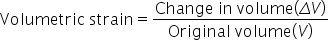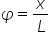# Strain

The ratio of change in configuration to the original configuration is called strain.

Being the ratio of two like quantities, it has no dimensions and units.

Strain are of three types :

(1) Linear strain : If the deforming force produces a change in length alone, the strain produced in the body is called linear strain or tensile strain.Linear strain in the direction of deforming force is called longitudinal strain and in a direction perpendicular to force is called lateral strain.

(2) Volumetric strain : If the deforming force produces a change in volume alone the strain produced in the body is called volumetric strain.(3) Shearing strain : If the deforming force produces a change in the shape of the body without changing its volume, strain produced is called shearing strain.

It is defined as angle in radians through which a plane perpendicular to the fixed surface of the cubical body gets turned under the effect of tangential force.Related Keywords